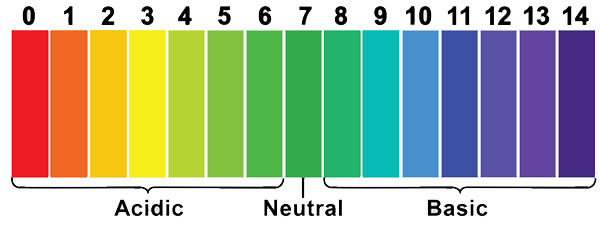# Two solutions X and Y have pH = 4 and pH = 8, respectively. Which solution will give alkaline reaction and which one acidic?

Two solutions X and Y have pH = 4 and pH = 8, respectively. Therefore, solution X will be acidic (pH less than 7) and solution Y will give alkaline reactions (pH greater than 7).

(Refer image for more details)Updated on: 10-Oct-2022

172 Views